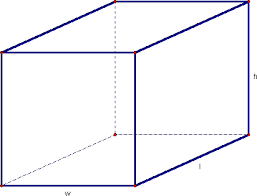## How to Calculate and Solve for the Width, Height, Length and Area of a Rectangular Prism | The Calculator EncyclopediaThe image above is a rectangular prism.

To compute the area of a rectangular prism requires three essential parameters which are the length, width and height of the rectangular prism.

A = 2wl + 2hl + 2hw

Where;
A = Area of the rectangular prism
l = Length of the rectangular prism
w = Width of the rectangular prism
h = Height of the rectangular prism

Let’s solve an example;
Find the area of a rectangular prism with a width of 10 cm, a height of 17 cm and a length of 14 cm.

This implies that;
l = Length of the rectangular prism = 14 cm
w = Width of the rectangular prism = 10 cm
h = Height of the rectangular prism = 17 cm

A = 2wl + 2hl + 2hw
A = 2 x 10 x 14 + 2 x 17 x 14 + 2 x 17 x 10
A = 280 + 476 + 340
A = 1096

Therefore, the area of the rectangular prism is 1096 cm2.

Calculating the Length of a Rectangular Prism using the Area, Width and Height of the Rectangular Prism.

l = A – 2hw / 2 (w + h)

Where;
l = Length of the rectangular prism
A = Area of the rectangular prism
w = Width of the rectangular prism
h = Height of the rectangular prism

Let’s solve an example;
Find the length of a rectangular prism with a width of 9 cm, a height of 12 cm and a Area of 250 cm2.

This implies that;
w = Width of the rectangular prism = 9 cm
h = Height of the rectangular prism = 12 cm
A = Area of the rectangular prism = 250 cm2

l = A – 2hw / 2 (w + h)
l = 250 – 2 x 12 x 9 / 2 (9 + 12)
l = 250 – 216 / 2 (21)
l = 34 / 42
l = 0.81

Therefore, the length of the rectangular prism is 0.81 cm.